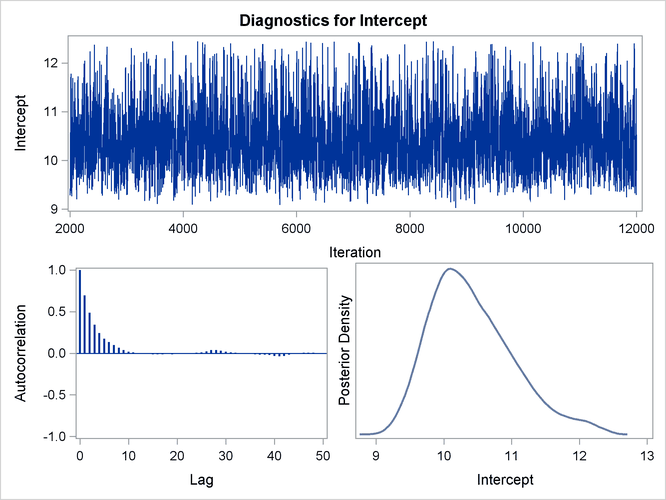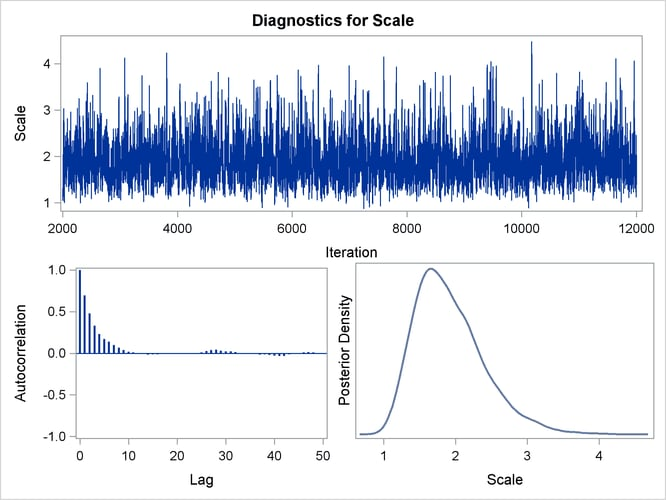Bayesian Analysis of Right-Censored Data

Nelson (1982) describes a study of the lifetimes of locomotive engine fans. This example shows how to use PROC LIFEREG to carry out a Bayesian analysis of the engine fan data. In this example, a lognormal distribution is used to model the engine lifetimes, but other survival time distributions, such as the Weibull, can also be used.

The following SAS statements create the SAS data set Fan. This data set contains a censoring indicator variable and right-censored survival times for the 70 locomotive engine fans in the study.

data Fan;
datalines;
450 0    460 1   1150 0   1150 0   1560 1
1600 0   1660 1   1850 1   1850 1   1850 1
1850 1   1850 1   2030 1   2030 1   2030 1
2070 0   2070 0   2080 0   2200 1   3000 1
3000 1   3000 1   3000 1   3100 0   3200 1
3450 0   3750 1   3750 1   4150 1   4150 1
4150 1   4150 1   4300 1   4300 1   4300 1
4300 1   4600 0   4850 1   4850 1   4850 1
4850 1   5000 1   5000 1   5000 1   6100 1
6100 0   6100 1   6100 1   6300 1   6450 1
6450 1   6700 1   7450 1   7800 1   7800 1
8100 1   8100 1   8200 1   8500 1   8500 1
8500 1   8750 1   8750 0   8750 1   9400 1
9900 1  10100 1  10100 1  10100 1  11500 1
;


Some of the fans had not failed at the time the data were collected, and the unfailed units have right-censored lifetimes. The variable Lifetime represents either a failure time or a censoring time. The variable Censor is equal to 0 if the value of Lifetime is a failure time, and it is equal to 1 if the value is a censoring time.

The following SAS statements specify a Bayesian analysis that uses a lognormal model for the engine lifetimes. There are no covariates, so the model is an intercept-only model. The OUTPOST= option saves the samples from the posterior distribution in the SAS data set Post for further processing.

ods graphics on;
proc lifereg data=Fan;
model Lifetime*Censor( 1 )= / dist=lognormal;
bayes seed=1  outpost=Post;
run;
ods graphics off;


The SEED= option is specified to maintain reproducibility; no other options are specified in the BAYES statement. By default, a uniform prior distribution is assumed for the intercept coefficient. The uniform prior is a flat prior on the real line with a distribution that reflects ignorance of the location of the parameter, placing equal probability on all possible values the regression coefficient can take. Using the uniform prior in the following example, you would expect the Bayesian estimates to resemble the classical results of maximizing the likelihood. If you can elicit an informative prior on the regression coefficients, you should use the COEFFPRIOR= option to specify it. A default noninformative gamma prior is used for the lognormal scale parameter.

You should make sure that the posterior distribution samples have achieved convergence before using them for Bayesian inference. If you do not specify additional options, PROC LIFEREG produces by default three convergence diagnostics: autocorrelations of the posterior sample, effective sample size, and the Geweke statistic. See the section Assessing Markov Chain Convergence in Chapter 7: Introduction to Bayesian Analysis Procedures, for information about assessing the convergence of the chain of posterior samples. Trace plots, posterior density plots, and autocorrelation function plots that are created using ODS Graphics are also provided for each parameter. See the section Visual Analysis via Trace Plots in Chapter 7: Introduction to Bayesian Analysis Procedures, for help in interpreting these plots.

The Analysis of Maximum Likelihood Parameter Estimates table in Figure 55.6 summarizes maximum likelihood estimates of the lognormal intercept and scale parameters.

Figure 55.6: Maximum Likelihood Estimates from the LIFEREG Procedure

The LIFEREG Procedure

Bayesian Analysis

Analysis of Maximum Likelihood Parameter Estimates
Parameter DF Estimate Standard Error 95% Confidence Limits
Intercept 1 10.1432 0.5211 9.1219 11.1646
Scale 1 1.6796 0.3893 1.0664 2.6453

Since no prior distribution for the intercept was specified, the default uniform improper distribution shown in the Uniform Prior for Regression Coefficients table in Figure 55.7 is used.

Noninformative prior distributions are appropriate if you have no prior knowledge of the likely range of values of the parameters, and if you want to make probability statements about the parameters or functions of the parameters. Refer, for example, to Ibrahim, Chen, and Sinha (2001) for more information about choosing prior distributions.

The default noninformative gamma prior distribution for the lognormal scale parameter is shown in the Independent Prior Distributions for Model Parameters table in Figure 55.7.

Figure 55.7: Noninformative Prior Distributions

The LIFEREG Procedure

Bayesian Analysis

Uniform Prior for Regression
Coefficients
Parameter Prior
Intercept Constant

Independent Prior Distributions for Model Parameters
Parameter Prior Distribution Hyperparameters
Scale Gamma Shape 0.001 Inverse Scale 0.001

By default, posterior mode estimates of the model parameters are used as the starting value for the simulation. These are listed in the Initial Values of the Chain table in Figure 55.8.

Figure 55.8: Markov Chain Initial Values

Initial Values of the Chain
Chain Seed Intercept Scale
1 1 10.0501 1.59544

Summary statistics for the posterior sample are displayed in the Fit Statistics, Descriptive Statistics for the Posterior Sample, Interval Statistics for the Posterior Sample, and Posterior Correlation Matrix tables in Figure 55.9. Since noninformative prior distributions were used, these results are consistent with the maximum likelihood estimates shown in Figure 55.6.

Figure 55.9: Posterior Sample Summary Statistics

Fit Statistics
DIC (smaller is better) 87.245
pD (effective number of parameters) 1.823

The LIFEREG Procedure

Bayesian Analysis

Posterior Summaries
Parameter N Mean Standard
Deviation
Percentiles
25% 50% 75%
Intercept 10000 10.4196 0.6172 9.9670 10.3259 10.7959
Scale 10000 1.9196 0.4809 1.5675 1.8476 2.1931

Posterior Intervals
Parameter Alpha Equal-Tail Interval HPD Interval
Intercept 0.050 9.4477 11.8994 9.3216 11.6752
Scale 0.050 1.1906 3.0570 1.1104 2.8834

Posterior Correlation Matrix
Parameter Intercept Scale
Intercept 1.0000 0.8297
Scale 0.8297 1.0000

By default, PROC LIFEREG computes three convergence diagnostics: the lag1, lag5, lag10, and lag50 autocorrelations; the Geweke diagnostic; and the effective sample size. These are displayed in Figure 55.10. There is no indication that the Markov chain has not converged. See the section Assessing Markov Chain Convergence in Chapter 7: Introduction to Bayesian Analysis Procedures, for more information about convergence diagnostics and their interpretation.

Figure 55.10: Posterior Sample Summary Statistics

The LIFEREG Procedure

Bayesian Analysis

Posterior Autocorrelations
Parameter Lag 1 Lag 5 Lag 10 Lag 50
Intercept 0.6973 0.1765 0.0190 -0.0017
Scale 0.6955 0.1713 0.0172 -0.0002

Geweke Diagnostics
Parameter z Pr > |z|
Intercept -0.9183 0.3585
Scale -0.9233 0.3559

Effective Sample Sizes
Parameter ESS Autocorrelation
Time
Efficiency
Intercept 1772.8 5.6408 0.1773
Scale 1805.0 5.5400 0.1805

Summary statistics of the posterior distribution samples are produced by default. However, these statistics might not be sufficient for carrying out your Bayesian inference. The samples from the posterior distribution saved in the SAS data set Post created with the OUTPOST= option can be used for further analysis. Trace, autocorrelation, and density plots for the three model parameters shown in Figure 55.11 and Figure 55.12 are useful in diagnosing whether the Markov chain of posterior samples has converged. These plots show no evidence that the chain has not converged. See the section Visual Analysis via Trace Plots in Chapter 7: Introduction to Bayesian Analysis Procedures, for more information about interpreting these types of diagnostic plots.

Figure 55.11: Diagnostic PlotsFigure 55.12: Diagnostic PlotsThe fraction failing in the first 8000 hours of operation might be a quantity of interest. This kind of information could be useful, for example, in determining whether to improve the reliability of the engine components due to warranty considerations. The following SAS statements compute the mean and percentiles of the distribution of the fraction failing in the first 8000 hours from the posterior sample data set Post:

data Prob;
set Post;
Frac = ProbNorm(( log(8000) - Intercept ) / Scale );
label Frac= 'Fraction Failing in 8000 Hours';
run;

proc means data = Prob(keep=Frac) n mean p10 p25 p50 p75 p90;
run;


The mean fraction of failures in the first 8000 hours, shown in Figure 55.13, is about 0.24, which could be used in further analysis of warranty costs. The 10th percentile is about 0.16 and the 90th percentile is about 0.32, which gives an assessment of the probable range of the fraction failing in the first 8000 hours.

Figure 55.13: Fraction Failing in 8000 Hours

The MEANS Procedure

Analysis Variable : Frac Fraction Failing in 8000 Hours
N Mean 10th Pctl 25th Pctl 50th Pctl 75th Pctl 90th Pctl
10000 0.2381467 0.1628591 0.1953691 0.2336756 0.2766051 0.3190883